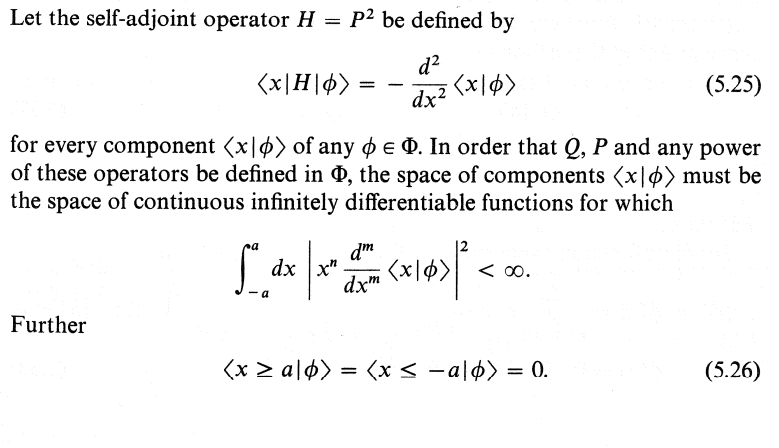# (Solved): Let P2 be the operator defined by Equation (5.25) and the operator defined by ...???????

Let P2 be the operator defined by Equation (5.25) and the operator defined by

With the same requirements for the functions . Show that as a consequence of (5.26) P and P2 are self-adjoint operators. Are P and P2 self-adjoint operators if (5.26) is not fulfilled?

Let the self-adjoint operator be defined by for every component of any . In order that and any power of these operators be defined in , the space of components must be the space of continuous infinitely differentiable functions for which Further

We have an Answer from Expert